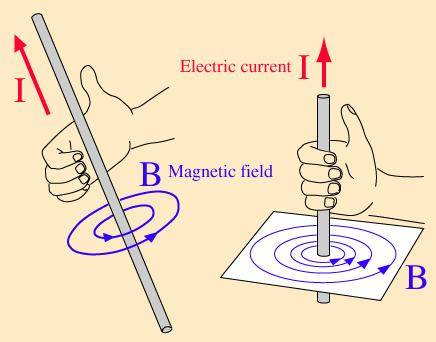# Artificial Magnetic Fields — Electromagnetism (Physics Gr12)

## Homework Statement

Two parallel conductors each carry 10 A of current in the same direction.
a) What is the magnetic field strength at the midpoint between these wires? (answer = 0T)
b) What is the field strength at the same point if the current ran in opposite directions? (answer = 4.0x10^-4T)

I am very confused and any help would be greatly appreciated

CWatters
Homework Helper
Gold Member
Are you familiar with the right hand rule..Make some drawings of the magnetic field with two wires.

Yes I am familiar with all three of them

CWatters